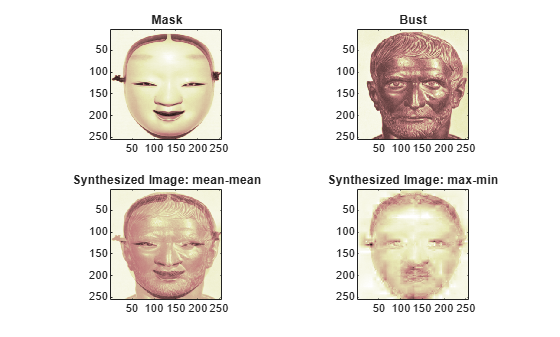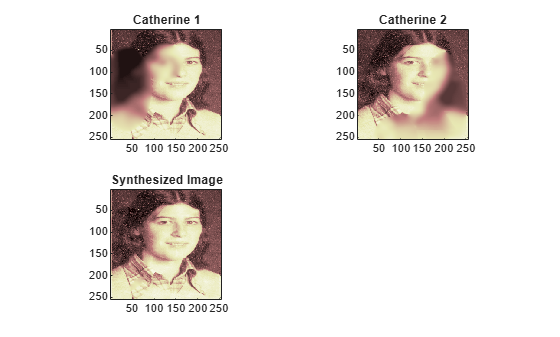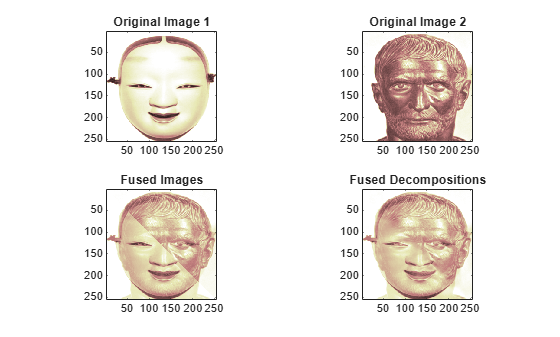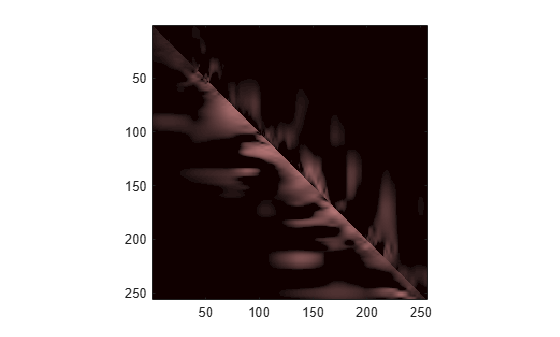# wfusimg

Fusion of two images

## Syntax

``xfus = wfusimg(x1,x2,wname,level,afusmeth,dfusmeth)``
``[xfus,txfus,tx1,tx2] = wfusimg(x1,x2,wname,level,afusmeth,dfusmeth)``
``[___] = wfusimg(___,'plot')``

## Description

The principle of image fusion using wavelets is to merge the wavelet decompositions of the two original images using fusion methods applied to approximations coefficients and details coefficients.

example

````xfus = wfusimg(x1,x2,wname,level,afusmeth,dfusmeth)` returns the fused image `xfus` obtained by fusion of the two original images `x1` and `x2`.```
````[xfus,txfus,tx1,tx2] = wfusimg(x1,x2,wname,level,afusmeth,dfusmeth)` also returns three wavelet decomposition tree objects associated with `xfus`, `x1`, and `x2`, respectively.```
````[___] = wfusimg(___,'plot')` plots the objects `txfus`, `tx1`, and `tx2`. This syntax can be used with any of the previous syntaxes.```

## Examples

collapse all

This example shows how to fuse two images to create a new image.

```load mask x1 = X; load bust x2 = X;```

Merge the two images from level 5 wavelet decompositions using the `db2` wavelet. Perform the fusion by taking the mean for both approximations and details.

```wv = 'db2'; lv = 5; xfusmean = wfusimg(x1,x2,wv,lv,'mean','mean');```

Merge the two images again, but this time perform the fusion by taking the maximum of the approximations and the minimum for the details.

`xfusmaxmin = wfusimg(x1,x2,wv,lv,'max','min');`

Plot the original and fused images.

```subplot(2,2,1) image(x1) axis square title('Mask') subplot(2,2,2) image(x2) axis square title('Bust') subplot(2,2,3) image(xfusmean) axis square title('Synthesized Image: mean-mean') subplot(2,2,4) image(xfusmaxmin) axis square title('Synthesized Image: max-min') colormap(map)```This example shows how to restore an image from two fuzzy versions of an original image.

Load two fuzzy versions of an original image.

```load cathe_1 x1 = X; load cathe_2 x2 = X;```

Merge the two images from level 5 wavelet decompositions using the `smy4` wavelet. Perform the fusion by taking the maximum of the absolute value of the coefficients for both approximations and details.

```wv = 'sym4'; lv = 5; xfus = wfusimg(x1,x2,wv,lv,'max','max');```

Plot the original and fused images.

```subplot(2,2,1) image(x1) axis square title('Catherine 1') subplot(2,2,2) image(x2) axis square title('Catherine 2') subplot(2,2,3) image(xfus) axis square title('Synthesized Image') colormap(map)```This example shows how to fuse two images using a user-defined fusion method.

Load two images of the same size.

```load mask a = X; load bust b = X;```

Define the fusion method and call the fusion function `helperUserFusion`. The source code for `helperUserFusion` is listed in the appendix.

`fus_method = struct('name','userDEF','param','helperUserFusion');`

Merge the images twice with the user-defined method. First use `wfusmat`, which fuses the images themselves and not their wavelet decompositions. Then use `wfusimg`, which fuses the wavelet decompositions.

```c = wfusmat(a,b,fus_method); d = wfusimg(a,b,'db4',5,fus_method,fus_method);```

Plot the original and fused images.

```subplot(2,2,1) image(a) title('Original Image 1') axis square subplot(2,2,2) image(b) title('Original Image 2') axis square subplot(2,2,3) image(c) title('Fused Images') axis square subplot(2,2,4) image(d) title('Fused Decompositions') axis square colormap(pink(220))```Visualize the differences between the merged images.

```figure image(c-d) axis square colormap(pink(220))```Appendix

helperUserFusion

If you want to try a different user-defined fusion method, edit the file helpUserFusion.m, which is located in the same folder as this example.

```function c = helperUserFusion(A,B) % This function is in support of the wavelet fusion examples only. It may % change or be removed in a future release. % create an upper triangular logical array the same size as A. d = logical(triu(ones(size(A)))); % set a threshold t = 0.3; c = A; % set the upper triangular portion of the output to a blend of A and B c(d) = t*A(d)+(1-t)*B(d); % set the lower triangular portion of the output to a different blend of A % and B c(~d) = t*B(~d)+(1-t)*A(~d); end ```

## Input Arguments

collapse all

Images to merge, specified as real-valued 2-D matrices or real-valued 3-D arrays. If specified as 3-D arrays, `x1` and `x2` are assumed to be color images in the RGB color space and the third dimension of the arrays must be 3.

The images `x1` and `x2` must be the same size. To resize the images, use `wextend` or `imresize`.

Wavelet used to create the wavelet decomposition, specified as a character vector or string scalar. The wavelet must be orthogonal or biorthogonal and recognized by `wfilters`.

Wavelet decomposition level, specified as a positive integer.

Fusion methods for approximations and details, respectively, each specified either as a structure array or as one of the values listed here. The approximation and details are merged element-wise.

`afusmeth`Description
`'max'`

Maximum

`'min'`

Minimum

`'mean'`

Mean

`'img1'`

First element

`'img2'`Second element
`'rand'`Random element

When specified as a structure array, the structure has the form `struct('name',nameMETH,'param',paramMETH)` where `nameMETH` can be one of the values listed here.

`nameMETH`Description
`'linear'`
`'UD_fusion'`Up-down fusion
`'DU_fusion'`Down-up fusion
`'RL_fusion'`Right-left fusion (column-wise fusion)
`'LR_fusion'`Left-right fusion (column-wise fusion)
`'UserDEF'`User-defined fusion

For the description of these options and the `paramMETH` parameter, see `wfusmat`.

Example: `afusmeth = struct('name','linear','param',0.3)`

Data Types: `double` | `struct`

## Output Arguments

collapse all

Fused image, returned as a real-valued 2-D matrix or a real-valued 3-D array. The fused image `xfus` has the same size as `x1` and `x2`.

Wavelet decomposition trees associated with `xfus`, `x1`, and `x2`, respectively, returned as `wdectree` objects.

Example: `plot(txfus)` plots the object in a GUI tool that you can use to inspect the tree.

 de Zeeuw, P. M. "Wavelet and image fusion." CWI, Amsterdam, March 1998. `https://groups.google.com/d/msg/comp.soft-sys.matlab/AjqIENmx1Z4/5g7QDFrZvWMJ`

 Li, H., B. S. Manjunath, and S. K. Mitra. "Multisensor Image Fusion Using the Wavelet Transform." Graphical Models and Image Processing. Volume 57, Issue 3, May 1995, pp. 235–245.

 Misiti, M., Y. Misiti, G. Oppenheim, and J.-M. Poggi. Les ondelettes et leurs applications. France: Hermes Science/Lavoisier, 2003.